# Subobject

(diff) ← Older revision | Latest revision (diff) | Newer revision → (diff)

of an object in a category

A concept analogous to the concept of a substructure of a mathematical structure. Let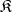be any category and letbe a fixed object in. In the class of all monomorphisms ofwith codomain (target), one may define a pre-order relation (the relation of divisibility from the right):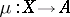precedes, or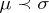, if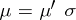for some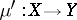. In fact, the morphism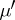is uniquely determined becauseis a monomorphism. The pre-order relation induces an equivalence relation between the monomorphisms with codomain: The monomorphismsandare equivalent if and only ifand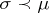. An equivalence class of monomorphisms is called a subobject of the object. A subobject with representativeis sometimes denoted by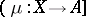or by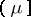. It is also possible to use Hilbert's-symbol to select representatives of subobjects ofand consider these representatives as subobjects. In the categories of sets, groups, Abelian groups, and vector spaces, a subobject of any object is defined by the imbedding of a subset (subgroup, subspace) in the ambient set (group, space). However, in the category of topological spaces, the concept of a subobject is wider than that of a subset with the induced topology.

The pre-order relation between the monomorphisms with codomaininduces a partial order relation between the subobjects of:if. This relation is analogous to the inclusion relation for subsets of a given set.

If the monomorphismis regular (cf. Normal monomorphism), then any monomorphism equivalent to it is also regular. One can therefore speak of the regular subobjects of any object. In particular, the subobject represented by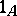is regular. In categories with zero morphisms one similarly introduces normal subobjects. Ifpossesses a bicategory structure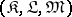, then a subobjectof an objectis called admissible (with respect to this bicategory structure) if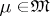.

The notationused in this article (and elsewhere in this Encyclopaedia) is not standard. Most authors do not bother to distinguish notationally between a subobject and a monomorphism which represents it.